# Matrix Exponentiation

## 1. What is Matrix Exponentiation

The matrix exponential plays an important role in the solution of systems of ordinary differential equations. It is a faster method that can be used to calculate the nth element of a series defined by a recurrence relation. This is one of the most used techniques in competitive programming.

For example,

In Fibonacci series problems, where we have to find the value of f(n) which is n’th Fibonacci number. When the value of n is sufficiently small, f(n) can be found by simple recursion F(n)=f(n-1)+f(n-2)

But, in the cases where the value of n is large, and the problem says

```{`
given 0< n <1000000000
find f(n) % 99999
`}```

The simple recursion method cannot be used also the dynamic programming will fail.

## 2. Matrix Exponential Algorithm

Now, let us see how matrix exponentiation can help us to represent recurrence relations.

### 2.1 RECURSIVE RELATIONS

The Fibonacci series is a sequence of numbers in which the first number is 0, the second number is 1 and all subsequent numbers are determined using the formula: f(n) = f(n-1) + f(n-2)

Such equation, in which one term of a sequence is defined using the previous terms i.e. value of the next term is a function of the previous term, is called a Recurrence relation.

A linear recurrence equation of degree k or order k is a recurrence equation which is in the format

```{`
X(n)=A1x(n−1) + A2x(n−1) + A3x(n−1) + …Akx(n) − kx(n)
= A1x(n−1) + A2x(n−1) + A3x(n−1) + …Akx(n−k)
`}```

(An is a constant and Ak≠0Ak≠0) on a sequence of numbers as a first-degree polynomial.

We can take the sequence defined by the recurrence relation a(n)= a(n−1) + 2, and a(0) = 1.

```{`
• The terms of the sequence are 1, 3, 5, 7, 9, ….
• A solution is a(n)=2n−1.
or
f(n)=f(n-3) + f(n-2) + f(n-1) which is an example of Tribonacci series
or
f(n)=3*f(n-1) + 7*f(n-2) an arbitrary example
are all recurrence relations.
`}```

### 2.2 REPRESENTING RECURSIVE RELATIONS USING MATRIX EXPONENTIAL

We can represent a recursive relation using a matrix M which can take us to the desired state from a set of known states.

Given, p states of a recurrence relation. Now, let us find the (p+1)th state of the recursive relation.

Let R be a p x p matrix, We have to create a matrix A:[p x 1] matrix from the known states of the recurrence relation given previously.

i.e. R is a p x p matrix & A is a p x 1 matrix
After building matrix A, let us get a matrix B:[p x 1] which will represent the set of next states
i.e. R x A = B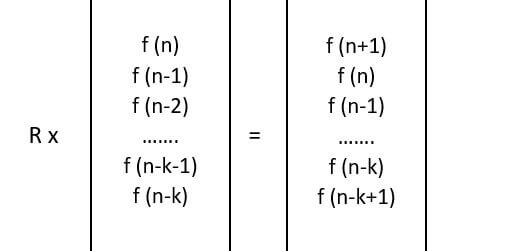Hence, once matrix R is designed, then matrix B can be found easily and the recurrence relation can be represented using the matrix designed as above.

## 3. Matrix Exponential Implementation

Matrix Exponential can be implemented by using the following function in our code.

1. Multiplying two matrices of appropriate size
2. Creating an identity matrix In;
3. Raising a matrix to r-th power using fast exponentiation.

The matrix should be stored as well. The easiest approach is to store matrices as 2D-arrays:

```{`
int matrix1 
int matrix2 
`}```

Now, let us implement Matrix Exponential using some recurrence relation.
Given, a recurrence relation, as follows

```{`
f(n) =  f(n-1) + f(n-2)
So, f(n+1) =  f(n) + f(n-1)
`}```

The exponential matrix can be used to represent the recurrence relation f(n) i.e. value of f(n+1) when value of f(n) and f(n-1) are given.

Matrix A and B can be represented as,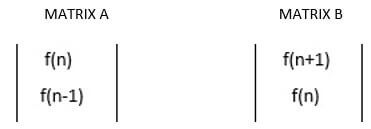Now , we have to design matrix M ( 2X2) such that,
M X A = B
Now, let us revise matrix multiplications
The product C of two matrices A and B is defined as
Cik = (Aij) (Bjk)

where j is summed over for all possible values of i and k and the notation above uses the Einstein summation convention. The implied summation over repeated indices without the presence of an explicit sum sign is called Einstein summation, and is commonly used in both matrix and tensor analysis. Therefore, in order for matrix multiplication to be defined, the dimensions of the matrices must satisfy

(n x m) (m x p)=(n x p)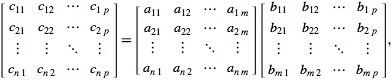Where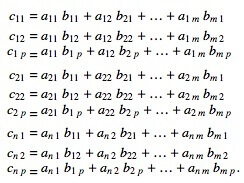The matrix A contains f(n) and f(n-1) and matrix B contains f(n+1) and f(n). The first element of matrix B is f(n+1) which can be computed as f(n) + f(n-1).

Since,
M ( a, b) X A (f(n)) = B(f(n+1))

The value of 1st row of M can be computed as [1 1].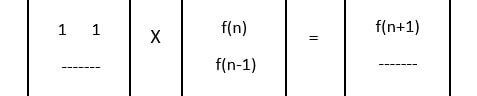Similarly, the value of second row of matrix M can be computed which is [1 0]
Since,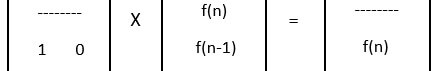In this way, the value of matrix M of size 2x2 could be found.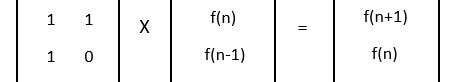## 4. Matrix Exponential Code in C++

Below is the C++ implementation of the Matrix Exponentiation.

AIM:
To find value of f(n) where f(n) is defined as
f(n) = f(n-1) + f(n-2) + f(n-3),
Where n >= 3 and f(0)=0, f(1)=1, f(2)=1

```{`
AIM:
To find value of f(n) where f(n) is defined as
f(n) = f(n-1) + f(n-2) + f(n-3),
Where n >= 3 and f(0)=0, f(1)=1, f(2)=1

PROGRAM:
#include
using namespace std;

// Function to multiply two matrices a and b.
void multiply(int a, int b)
{
// Matrix to store elements at the time of matrix multiplication
int mul;
for (int i = 0; i < 3; i++)
{
for (int j = 0; j < 3; j++)
{
mul[i][j] = 0;
for (int k = 0; k < 3; k++)
mul[i][j] += a[i][k]*b[k][j];
}
}

// storing the value of multiplication in matrix a
for (int i=0; i<3; i++)
for (int j=0; j<3; j++)
a[i][j] = mul[i][j];
}

// Function to compute F raise to power n-2.
int power(int F, int n)
{
int M = {1,1,1}, {1,0,0}, {0,1,0};

if (n==1)
return F + F;

power(F, n/2);

multiply(F, F);

if (n%2 != 0)
multiply(F, M);

return F + F ;
}

// Function to find nth term of series
int findNthTerm(int n)
{
int F = {1,1,1}, {1,0,0}, {0,1,0} ;

return power(F, n-2);
}

// Main function
int main()
{
int n = 5;

cout << "F(5) is " << findNthTerm(n);

return 0;
}

OUTPUT:
F(5) is 7

TIME COMPLEXITY:
O(log n)
`}```

## 5. Applications of Matrix Exponential

5.1 Finding the nth element of Fibonacci Series

Fibonacci numbers can be defined using f(n) as follows:

1. F0 = F1 = 1;
2. Fi = Fi - 1 + Fi - 2 for i ≥ 2.

We want to find FN modulo 1000000007, where N can be up to 1018.

Pseudo code:

```{`
function fibonacci_exponentiation(N):
if N <= 1:
return 1
initial = (1, 1)
exp = matrix_power_with_modulo(M, N - 1, 1000000007) // assuming we’ve defined M
return (initial * exp) modulo 1000000007
`}```

### 5.2 Finding Nth element of Linear Recurrent Sequence

The sequence A satisfies two properties:

1. Ai = c1 * Ai- 1 + c2 * Ai- 2 + … + ck * Ai - k for i ≥ k (c1, c2 …, ck are given integers);
2. A0 = a0, A1 = a1, …, Ak- 1 = ak - 1 (a0, a1, …, ak - 1 are given integers). We need to find AN modulo 1000000007, when N is up to 1018 and k up to 50.

Pseudo code:

```{`
dp[a] = dp[b] = … = dp[z] = 1
for n = 2..L:
for last_letter = a..z:
for next_letter = a..z:
if banned[last_letter][next_letter] != 0:
dp[n + 1][next_letter] += dp[n][last_letter]
`}```

### 5.3 Finding the sum of Fibonacci numbers up to N

Let’s go back to Fibonacci numbers:

1. F0 = F1 = 1;
2. Fi = Fi - 1 + Fi - 2 for i ≥ 2.

Introduce a new sequence: Pi = F0 + F1 + … + Fi (i.e. sum of first i Fibonacci numbers). The problem is: find PN modulo 1000000007 for N up to 1018.

Pseudo code:

To find sum of first N numbers of any recurrent linear sequence Ai,
introduce the “sum sequence” Pi = A0 + A1 + … + Ai.
Put this sequence into the initial vector.
Then find the matrix that gives you Pi + 1, based on Pi and some A’s.

### 5.4 Computing multiple linear recurrent sequences at once

Given integer n ≥ 106, find the number of pairs of integers (x, y) such that:

1. 0 ≤ x < 2n;
2. 0 ≤ y < 2n;
3. Bitwise OR of x and y is not equal to x;
4. Bitwise OR of x and y is not equal to y.

Pseudo code:

let dpi be the answer for n = i.
Then it can be shown that:
dpi = dpi - 1 * 4 + gi - 1 * 2 for i > 1,
dp1 = 0,
where sequence g satisfies:
gi = gi - 1 * 3 + 2i - 1 for i > 1,
g1 = 1.

### 5.5 Solving dynamic programming problems with fixed linear transitions

Count the number of strings of length L with lowercase English letters, if some pairs of letters can not appear consequently in those strings. L is up to 107, there are up to 100 inputs.

Pseudo code:

Let dp[n][last_letter] be the number of valid strings of length n, which have last letter equal to last_letter. Then we can recalculate dp in order of increasing n:

```{`
dp[a] = dp[b] = … = dp[z] = 1
for n = 2..L:
for last_letter = a..z:
for next_letter = a..z:
if banned[last_letter][next_letter] != 0:
dp[n + 1][next_letter] += dp[n][last_letter]
`}```

### BIBLIOGRAPHY

 Mike Koltsov, August,2016. Matrix exponentiation. https://www.hackerearth.com/practice/notes/matrix-exponentiation-1/